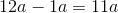# AP Statistics : How to find the range for a set of data

## Example Questions

### Example Question #1 : How To Find The Range For A Set Of Data

Six homes are for sale and have the following dollar values in thousands of dollars:

535

155

305

720

315

214

What is the range of the values of the six homes?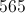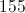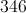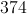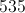Explanation:

The range is the simplest measurement of the difference between values in a data set.  To find the range, one simply subtracts the lowest value from the greatest value, ignoring the others.  Here, the lowest value is 155 and the greatest is 720.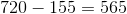### Example Question #2 : How To Find The Range For A Set Of Data

Alice recorded the outside temperature at noon each day for one week. These were the results.

Monday: 78

Tuesday: 85

Wednesday: 82

Thursday: 84

Friday: 82

Saturday: 79

Sunday: 80

What is the range of temperatures?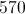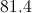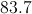Explanation:

The range is the simplest measurement of the difference between values in a data set.  To find the range, simply subtract the lowest value from the greatest value, ignoring the others.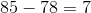### Example Question #1 : How To Find The Range For A Set Of Data

Find the range for the set.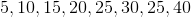Explanation:

To find the range, subtract the minimum value from the maximum value

minimum:maximum:So,

maximum - minimum =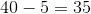### Example Question #4 : How To Find The Range For A Set Of Data

A business tracked the number of customer calls received over a period of five days.  What was the range of customer calls received daily?

Day 1: 57

Day 2: 63

Day 3: 48

Day 4: 49

Day 5: 59Explanation:

The range is the simple measurement of the difference between values in a dataset.

To find the range, simply subtract the lowest value from the greatest value, ignoring the others.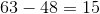### Example Question #1 : How To Find The Range For A Set Of Data

Find the range for the set of data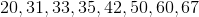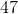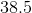Explanation:

The range is equal to the absolute difference between the minimum and maximum value.

We find the range to be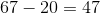### Example Question #6 : How To Find The Range For A Set Of Data

Letbe a positive integer.

What is the range of the set.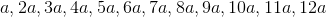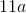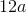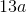Explanation:

To find the range, subtract the minimum value from the maximum value

minimum:maximum:So,

maximum - minimum =